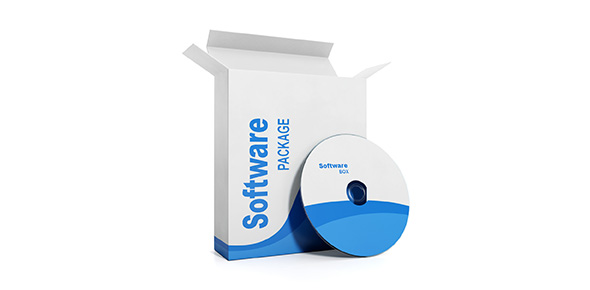# Nmit-c Aptitude Test Demo

5 Questions | Total Attempts: 73Settings• 1.
Int a=5,b=7;b=a++;a=a+++b++;printf("%d",a);
• A.

12

• B.

14

• C.

13

• D.

15

• 2.
Int x=40;main(){int x=20;printf("\n%d",x);}
• A.

20

• B.

40

• C.

60

• D.

Null

• 3.
What would be the output of the following program?main(){extern int i;i=20;printf("%d",sizeof(i));}
• A.

2

• B.

4

• C.

Would vary from compiler to compiler

• D.

Error, i undefined

• 4.
What would be the output of following program?main(){static int a;int i=0;a[i]=i++;printf("\n%d %d %d",a,a,i);}
• A.

0 0 0

• B.

1 1 1

• C.

0 0 1

• D.

1 1 2

• 5.
Point out the error,if any, in the while loop.main(){int i=1;while( ){printf("%d",i++);if(i>10)break;}}
• A.

The condition in the while loop is a must.

• B.

There should be at least a semicolon in the while( )

• C.

The while loop should be replaced by a for loop

• D.

No error

Related TopicsBack to top